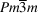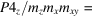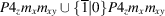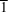International
Tables for
Crystallography
Volume A
Space-group symmetry
Edited by M. I. Aroyo

International Tables for Crystallography (2016). Vol. A, ch. 1.7, pp. 138-139

Section 1.7.3.2. The symmetry of domain walls

D. B. Litvinc and V. Kopskýd

1.7.3.2. The symmetry of domain walls

| top | pdf |

The cubic phase of barium titanate BaTiO3, of symmetry type, undergoes a phase transition to a tetragonal phase of symmetry typewhich can give rise to six distinct single-domain states (Janovec et al., 2004). This is represented in Fig. 1.7.3.2, where at the centre are four unit cells of the cubic phase with barium and titanium atoms represented by large and small filled circles, respectively, and oxygen atoms, which are located at the centre of each unit-cell face, as open circles. A cubic-to-tetragonal phase transition gives rise to atomic displacements represented by arrows, and to six single-domain states, four of which are depicted in the figure. The polar tetragonal symmetry of each of these tetragonal domain states is also shown.Figure 1.7.3.2 | top | pdf |At the centre is the structure of the cubic phase of barium titanate, BaTiO3, of symmetry type, surrounded by the structures of four of the six single-domain states of the tetragonal phase of symmetry type P4mm. All the diagrams are projections along the  direction. Arrows depict the atomic displacement amplitudes from their cubic-phase positions.

In determining the symmetry of a domain wall, we first construct a domain twin (Janovec & Přívratská, 2014): we choose two single-domain states, for this example the two in Fig. 1.7.3.2with symmetry P4zmxmxy, and construct a domain pair consisting of the superposition of these two single-domain states, see Fig. 1.7.3.3. The domain twin we choose to construct is obtained by passing a plane of orientation (010) through this domain pair at the origin and deleting from one side of the plane the atoms of one of the single-domain states, and the atoms of the second single-domain state from the other side of the plane, see Fig. 1.7.3.4. The plane is referred to as the central plane of the domain wall, and the atoms in and near this plane as the domain wall.Figure 1.7.3.3 | top | pdf |The domain pair of symmetry P4z/mzmxmxy consisting of the superposition of those two single-domain states of tetragonal symmetry P4zmxmxy shown in Fig. 1.7.3.2. The diagram is a projection along the  direction.

The symmetry of the central plane of the domain wall is determined from the symmetry of the domain pair and the scanning tables: The symmetry of the domain pair is the group of operations that either leaves both single-domain states invariant or simultaneously switches the two domain states. P4zmxmxy leaves both single-domain states invariant and the symmetry operation of spatial inversion switches the two single-domain states, see Fig. 1.7.3.3. Consequently, the domain-pair symmetry is. The symmetry of the central plane is determined from the scanning table for the space group P4z/mzmxmxy, the orientation orbit (010), the orientation of the domain wall and the linear orbit 0d, since the central plane of the wall passes through the origin (Volume E, 2010). The symmetry of the central plane is the sectional layer group pmxmzmy, where p denotes the lattice of translations in the x, 0, z plane.

Let n denote a unit vector perpendicular to the central plane of the domain wall; in this example n is in the  direction. The symmetry of the domain wall consists of:

 (1) all elements of the symmetry group of the central plane that leave n and both domain states invariant, i.e. in this example, all translations of p, 1 and mx; and (2) all elements of the symmetry group of the central plane that invert n and switch the domain states, i.e. in this example, 2x and.

The symmetry of the domain wall is then p2x/mx.

References

International Tables for Crystallography (2010). Vol. E, Subperiodic Groups, edited by V. Kopský & D. B. Litvin, 2nd ed. Chichester: John Wiley & Sons.
Janovec, V., Grocký, M., Kopský, V. & Kluiber, Z. (2004). On atomic displacements in 90° ferroelectric domain walls of tetragonal BaTiO3 crystals. Ferroelectrics, 303, 65–68.
Janovec, V. & Přívratská, J. (2014). Domain structures. In International Tables for Crystallography, Vol. D, Physical Properties of Crystals, edited by A. Authier, 2nd ed., ch. 3.4. Chichester: Wiley.